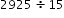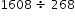# MAFS.6.NS.2.2Archived StandardExport Print
Fluently divide multi-digit numbers using the standard algorithm.

### Remarks

Fluency Expectations or Examples of Culminating Standards

Students fluently divide multi-digit numbers using the standard algorithm. This is the culminating standard for several years’ worth of work with division of whole numbers.
General Information
Subject Area: Mathematics
Domain-Subdomain: The Number System
Cluster: Level 1: Recall
Cluster: Compute fluently with multi-digit numbers and find common factors and multiples. (Additional Cluster) -

Clusters should not be sorted from Major to Supporting and then taught in that order. To do so would strip the coherence of the mathematical ideas and miss the opportunity to enhance the major work of the grade with the supporting clusters.

Date of Last Rating: 02/14
Status: State Board Approved - Archived
Assessed: Yes
Test Item Specifications

• Assessment Limits :
Items may only have 5-digit dividends divided by 2-digit divisors or 4-digit dividends divided by 2- or 3-digit divisors. Numbers in items are limited to non-decimal rational numbers.
• Calculator :

No

• Context :

No context

Sample Test Items (2)
• Test Item #: Sample Item 1
• Question:

An expression is shown.What is the value of the expression?

• Difficulty: N/A
• Type: EE: Equation Editor

• Test Item #: Sample Item 2
• Question:

An expression is shown.What is the value of the expression?

• Difficulty: N/A
• Type: EE: Equation Editor

## Related Courses

This benchmark is part of these courses.
1205010: M/J Grade 6 Mathematics (Specifically in versions: 2014 - 2015, 2015 - 2022, 2022 and beyond (current))
1205020: M/J Accelerated Mathematics Grade 6 (Specifically in versions: 2014 - 2015, 2015 - 2020, 2020 - 2022, 2022 and beyond (current))
1204000: M/J Foundational Skills in Mathematics 6-8 (Specifically in versions: 2014 - 2015, 2015 - 2022, 2022 and beyond (current))
7812015: Access M/J Grade 6 Mathematics (Specifically in versions: 2014 - 2015, 2015 - 2018, 2018 - 2022, 2022 and beyond (current))
7912110: Fundamental Explorations in Mathematics 1 (Specifically in versions: 2013 - 2015, 2015 - 2017 (course terminated))

## Related Access Points

Alternate version of this benchmark for students with significant cognitive disabilities.

## Related Resources

Vetted resources educators can use to teach the concepts and skills in this benchmark.

## Formative Assessments

Long Division - 1:

Students are asked to complete three long division problems (no remainders) to check for fluency with the standard division algorithm.

Type: Formative Assessment

Long Division - 3:

Students are asked to complete three long division problems (each with a remainder) to check for fluency with the standard division algorithm.

Type: Formative Assessment

Long Division - 2:

Students are asked to complete three long division problems (one with a remainder) to check for fluency with the standard long division algorithm.

Type: Formative Assessment

## Lesson Plans

Cracking the Code: A Division Challenge:

In this lesson, students will use their knowledge of division and division strategies to solve problems that have been written in a code where numbers replaced with symbols. The students are challenged to find out how the traditional algorithm strategy works and how it compares to other strategies they already know. Students are also charged with creating a list of mathematical tools that help make solving division problems easier.

Type: Lesson Plan

I want a cell phone:

Students develop a procedure to select a cell phone based on qualities they think their parents value in a cell phone. Students present their solution in writing to a marketing company, who wishes to use the results to market cell phones to parents of elementary students.

Type: Lesson Plan

Dividing Decimals Investigations:

In this introductory lesson, students test how the basic operations performed on the dividend and divisor affect the quotient of a pair of numbers. Students then conclude whether the results of their trials can be applied to solve problems with a decimal divisor.

Type: Lesson Plan

## Original Student Tutorial

MacCoder’s Farm Part 1: Declare Variables:

Explore computer coding on the farm by declaring and initializing variables in this interactive tutorial. You'll also get a chance to practice your long division skills.

Type: Original Student Tutorial

Reasoning about Multiplication and Division and Place Value, Part 2:

Students are asked to reason about and explain the placement of decimals in quotients.

Setting Goals:

The purpose of this task is for students to solve problems involving multiplication and division of decimals in the real-world context of setting financial goals. The focus of the task is on modeling and understanding the concept of setting financial goals, so fluency with the computations will allow students to focus on other aspects of the task.

Interpreting a Division Computation:

The purpose of this task is to use the computation shown below to find the products. Understanding of place value is necessary for successful completion.

## Student Center Activity

Students can practice answering mathematics questions on a variety of topics. With an account, students can save their work and send it to their teacher when complete.

Type: Student Center Activity

## Tutorial

Percent Word Problem:

Use long division to find the percent in this tutorial.

Type: Tutorial

## STEM Lessons - Model Eliciting Activity

I want a cell phone:

Students develop a procedure to select a cell phone based on qualities they think their parents value in a cell phone. Students present their solution in writing to a marketing company, who wishes to use the results to market cell phones to parents of elementary students.

## MFAS Formative Assessments

Long Division - 1:

Students are asked to complete three long division problems (no remainders) to check for fluency with the standard division algorithm.

Long Division - 2:

Students are asked to complete three long division problems (one with a remainder) to check for fluency with the standard long division algorithm.

Long Division - 3:

Students are asked to complete three long division problems (each with a remainder) to check for fluency with the standard division algorithm.

## Computer Science Original Student Tutorials

MacCoder’s Farm Part 1: Declare Variables:

Explore computer coding on the farm by declaring and initializing variables in this interactive tutorial. You'll also get a chance to practice your long division skills.

## Student Resources

Vetted resources students can use to learn the concepts and skills in this benchmark.

## Original Student Tutorial

MacCoder’s Farm Part 1: Declare Variables:

Explore computer coding on the farm by declaring and initializing variables in this interactive tutorial. You'll also get a chance to practice your long division skills.

Type: Original Student Tutorial

Reasoning about Multiplication and Division and Place Value, Part 2:

Students are asked to reason about and explain the placement of decimals in quotients.

Setting Goals:

The purpose of this task is for students to solve problems involving multiplication and division of decimals in the real-world context of setting financial goals. The focus of the task is on modeling and understanding the concept of setting financial goals, so fluency with the computations will allow students to focus on other aspects of the task.

## Student Center Activity

Students can practice answering mathematics questions on a variety of topics. With an account, students can save their work and send it to their teacher when complete.

Type: Student Center Activity

## Tutorial

Percent Word Problem:

Use long division to find the percent in this tutorial.

Type: Tutorial

## Parent Resources

Vetted resources caregivers can use to help students learn the concepts and skills in this benchmark.